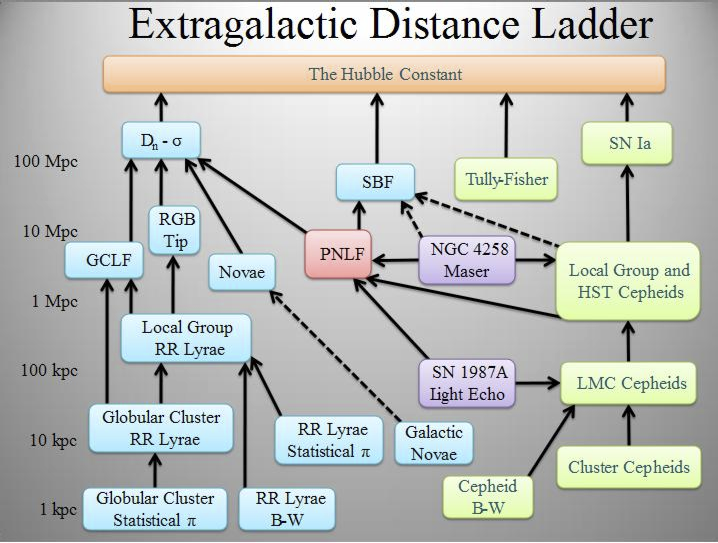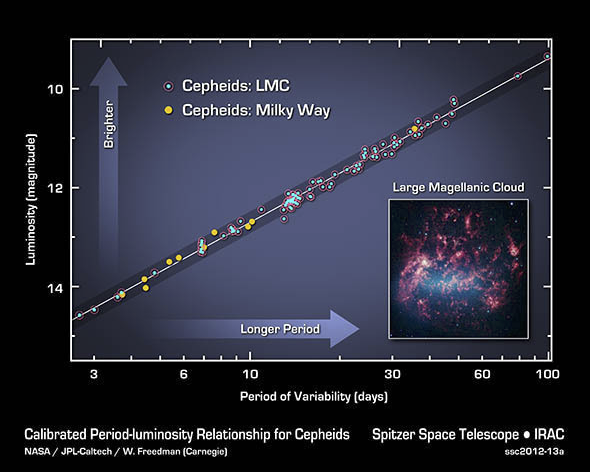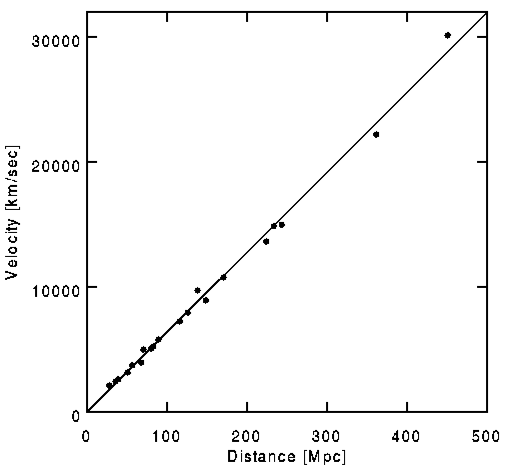## Section59.7Hubble's Law

The dual discoveries in 1912, Cepheid variables as standard candles by Henrietta Leavitt and red shifts of spiral nubulae by Vesto Slipher, were instrumental for a systematic study of distant objects. Edwin Hubble and Milton Humason used the relation between luminosity and period of Cepheids and the recessional speeds obtained from the redshifts and found the relation between redshifts and distance to galaxies, which is now known as the Hubble's Law.

Einstein's theory of gravitation, introduced in 1916, provided powerful tools for interpreting and understanding the meaning behind Hubble's law. In Einstein's theory, gravitation is treated as a geometric property of space and time. A model of universe based on a dynamic space, called the Freedman-LeMa\^itre -Robertson–Walker (FLRW) Model or the Hot Big Bang model, gave interpretation of the Hubble's law as due to the expansion of space itself.

### Subsection59.7.1Extragalactic Distances

The distances in the universe cover a very wide range, from about $\lt 10^{-15}$ m in nucleus of an atom to $14.0$ Gpc or $4\times 10^{26}$ m, the known size of the universe. Some of the representative sizes and distances of interest in Astronomy are given in Table 59.7.1

How do we measure these distances and how well do we know them? These are important questions of practical value. There are actually a variety of methods, that make up a distance ladder, which let us go from smaller distances to the enormous distances as illustrated Figure 59.7.2.Figure 59.7.2. Extragalactic Distance Ladder. Legend: (1) Light green boxes: Technique applicable to star-forming galaxies, (2) Light blue boxes: Technique applicable to Population II galaxies, (3) Light Purple boxes: Geometric distance technique, (4) Light Red box: The planetary nebula luminosity function technique is applicable to all populations of the Virgo Supercluster, (5) Solid black lines: Well calibrated ladder step, (6) Dashed black lines: Uncertain calibration ladder step. Credits: WilliamKF, Wikimedia Commons.

The nearby distances, which are less than about $1\text{ kpc}\text{,}$ can be obtained with great confidence using the Parallax method we have studied above. We can extend the use of Parallax method by combining it with the luminosity-period relation of Cepheid variables and extend the distance measurement to 10-20 Mpc, which takes us to a distance of Large Magellanic Cloud and Local Group of galaxies.We will discuss the method based on Cepheid variables in more detail below.

Next scale of extension is to $100\text{ Mpc}$ by using the relation between orbital speed of stars at the edge of a spiral galaxy and the luminosity of the galaxy, called the Tully-Fisher relation. Even farther distances are obtained by using the peak luminosity of type Ia supernova explosion, which is assumed to be same for all type I supernova, thus giving an absolute luminosity. The apparent luminosity is then compared to the assumed absolute luminosity to obtain the distance in a similar calculation used for Cepheids and RR Lyrae. The type Ia supernovae take us to $\sim$ 1 Gpc.

#### Subsubsection59.7.1.1Cepheids as standard candle

The brightness of most stars vary with time due to the internal dynamics of nuclear processes in the stars and the change in opacity of the stars. In Magellanic Clouds, Henriette Leavitt found 1777 variable stars. Of these, she noted that 25 were Cepheids in the Small Magellanic Cloud (SMC). Cepheids are super giant stars which have completed most of their thermonuclear fuel and pulsate due to instability of their gas cloud when radiation passes through them. The light curve of a prototype Cepheid star, the Delta Cephei, is shown in Figure 59.7.3.

When Leavitt organized the Cepheids according to their periods, she noticed that the stars with longer periods also corresponded to the stars with higher brightness. Since the Cepheids that Leavitt was studying at the time were in the same cluster of stars, she assumed that they were perhaps the same distance from the Earth. With this assumption, she was able to conclude that:

“Since the variables are probably at nearly the same distance from the Earth, their periods are apparently associated with their actual emission of light, as determined by their mass, density, and surface brightness.”

A current plot of luminosity (in units of magnitude) versus period of several Cepheids in Milky Way and Large Magellanic Cloud is shown in Figure 59.7.4. The linearity of the plot is very remarkable. To find the distance a cluster of stars we need only find a Cepheid in the cluster and measure its period ($T$) and average apparent brightness $m\text{,}$ which is the average of the maximum brightness and the minimum brightness. Now, we use the period $T$ in a plot similar to the plot in Figure 59.7.4 to determine the absolute magnitude $M$ of the Cepheid. The relation between the absolute magnitude and the apparent magnitude gives us the distance to the Cepheid and hence to the cluster of stars under study by Eq. (59.2.6).

\begin{equation*} m - M = 5.0\:\log_{10} \left( \frac{d\ [\text{pc}]}{10\: \text{pc}}\right). \end{equation*}Figure 59.7.4. The luminosity-period plot of Cepheids in Milky Way and Large Magellanic Cloud. Credits: NASA/JPL-Caltech/W. Freedman (Carnegie).

An astronomer observed a Cepheid star with a period of 25 days with apparent magnitude +20. What is the distance of the Cepheid?

Hint

Use luminosity-period plot.

$5,011\:\text{pc}\text{.}$

Solution

Looking up the luminosity-period plot we find that the absolute magnitude corresponding to the period of 25 days is $M=11.5\text{.}$ From $m - M = 5 \log_{10} d - 5$ with $d$ in pc, we get

\begin{equation*} 25 - 11.5 = 5 \log d - 5 \end{equation*}

Solving for $d$ gives

\begin{equation*} d = 10^{18.5/5}\:\text{pc} = 5,011\:\text{pc}. \end{equation*}

### Subsection59.7.2Recession Velocities

In 1912 Vesto Melvin Slipher reported the redshift of spectral lines from Andromeda galaxy. Throughout 1910s Slipher studied the spectra of several nubulae (which, at the time, were not known to be separate galaxies) at the Lowell Observatory, Arizona, USA. To determine the recession velocity of a star we first identify a characteristic spectral line for an element in the spectrum of the star, and then, compare the wavelength measured to the wavelength seen in an experiment in the lab. From the fractional difference we can deduce the speed of recession of the galaxy by using the Doppler formula as illustrated below.

If a star emits a light of wavelength $\lambda_0\text{,}$ the wavelength $\lambda$ detected at the Earth will be longer if Earth and star are moving away from each other and shorter if the Earth and the star are moving towards each other. When the measured wavelength is longer we say the light is red shifted and when it is shorter we say the light is blue shifted. For instance, a hydrogen emission spectrum in the lab where the hydrogen gas and the detector are at rest with respect to each other contains light of wavelengths

\begin{equation*} \text{Balmer lines:}\ \text{H}_{\alpha}\ 656.3\ \text{nm},\ \text{H}_{\beta}\ 486.1\ \text{nm},\ \text{H}_{\gamma}\ 434.1\ \text{nm},\ \text{H}_{\delta}\ 410.2\ \text{nm}. \end{equation*}

If a star is receding at a speed of 300 km/s these lines will appear at

\begin{equation*} \text{Red shifted Balmer lines:}\ \text{H}_{\alpha}^{\prime}\ 656.957\ \text{nm},\ \text{H}_{\beta}^{\prime}\ 486.586\ \text{nm},\ \text{H}_{\gamma}^{\prime}\ 434.534\ \text{nm},\ \text{H}_{\delta}^{\prime}\ 410.61\ \text{nm}. \end{equation*}

This effect is called Doppler effect. With a relative speed $v$ along the line of sight of the star from Earth, the Doppler effect of electromagnetic waves gives the following relation

\begin{equation} \frac{\lambda}{\lambda_0} = \sqrt{\frac{1+v/c}{1-v/c}}, \label{eq-rel-doppler-shift-1}\tag{59.7.1} \end{equation}

where $c$ is the speed of light. It is customary to define a dimensionless parameter $z$ that measures fractional change in the wavelength.

\begin{equation*} z = \frac{\lambda - \lambda_0}{\lambda_0}. \end{equation*}

Thus, the relativistic Doppler shift, Eq. (59.7.1), in terms of red shift becomes

\begin{equation} 1 + z = \sqrt{\frac{1+v/c}{1-v/c}}, \label{eq-rel-doppler-shift-1a}\tag{59.7.2} \end{equation}

Solving for $v$ we get

\begin{equation} \frac{v}{c} = \frac{z(2+z)}{1 + (1+z)^2}. \label{eq-rel-doppler-shift-2}\tag{59.7.3} \end{equation}

If $v \ll c\text{,}$ we get a much simpler formula,

\begin{equation*} v = z c.\ \ \ \ (v \ll c). \end{equation*}

These Doppler shifts were later interpreted as red shifts due to the cosmological expansion of space after Hubble discovered that the recession velocities correlated with the distance to the galaxies. We will examine this later interpretation below.

### Subsection59.7.3Hubble's Law

In 1920s, using Henrietta Leavitt's method of Cepheid variables, Edwin Hubble and Milton Humason of Mt. Wilson Observatory, USA deduced the distances to nubulae. They plotted the velocities of the nebulae obtained by Slipher based on the red shift of spectral lines from those nebulae against their distances. Although the plot had a considerable scatter but a general trend was observed that indicated that the nebulae that were further away had a higher recessional speed. Even Einstein was satisfied with the general conclusion from the data and visited Hubble at Mount Wilson Observatory, near Pasadena, California, in 1931. This result is called Hubble's law.

Figure 59.7.7 shows recessional velocity versus distance to a much further distance than was available to Hubble. Let $v$ be the recessional velocity and $D$ the distance to the galaxy, then Hubble's law says that

\begin{equation*} v = H_0 D, \end{equation*}

where the proportionality constant $H_0$ is called the Hubble's constant. The values based on the Wilkinson Microwave Anisotropy Probe (WMAP), a NASA Explorer mission launched in June 2001, has yielded a much more precise value of $H_0\text{.}$ With the assumption of a flat universe to be discussed below, the best value of $H_0$ was found to be

\begin{equation*} H_0 = 73.8\pm2.4\:\frac{\text{km/s}}{\text{Mpc}}. \end{equation*}Figure 59.7.7. Hubble's law: the recessional velocity of galaxies is proportional to distance. From Riess, Adam G., Press, William H., Kirshner, Robert P., Astrophysical Journal v.473, p.88.

The galaxy 8C1435+635 has a red shift $z=4.25\text{.}$ (a) How fast is the galaxy receding with respect to Earth? (b) How far away is the galaxy?

Hint

Solution

(a) Since $z \gt 1$ we cannot use the assumption $v \ll c\text{.}$ We need to use the relativistic formula, Eq. (59.7.3) to find $v\text{.}$

\begin{equation*} \frac{v}{c} = \frac{z(2+z)}{1 + (1+z)^2} = \frac{4.25 \times 6.25}{1 + 5.25^2} = 0.93. \end{equation*}

Multiplying by $c = 3\times 10^8$ m/s we find

\begin{equation*} v = 2.8 \times 10^{8}\:\text{m/s}. \end{equation*}

(b) Using the Hubble's law we can find the distance to the galaxy if we know its recession velocity.

\begin{equation*} D = \frac{v}{H_0} = \frac{2.8 \times 10^{8}\:\text{m/s}}{73.8\times 10^3\:\text{m/s per Mpc}} = 3.8 \times 10^3\:\text{Mpc}. \end{equation*}
TODO:

\​begin{exercise} Find the distances to galxies recessional velocities are (a) $\frac{c}{50}$, (b) $\frac{c}{10}$, (c) $\frac{c}{2}$? \end{exercise}

\​begin{exercise} The red shift of a galaxy is observed to be $z = 0.1$. (a) What is the recession speed of the galaxy? (b) How far away is the galaxy? \end{exercise}

\​begin{exercise} A distant galaxy has strong hydrogen spectral lines. The line for $H_\alpha$ is found to be at 7560 \AA rather than 6562.8 \AA seen from a hydrogen atom in the lab. (a) What is the red shift of the galaxy? (b) How fast is the galaxy receding? (c) How far is the galaxy from Earth? \end{exercise}

\​begin{exercise} A Cepheid star has period of 5 days and apparent brightness of +10. How far is the star? \end{exercise}

\​begin{exercise} Astronomers find that if all Type Ia supernovae were at a distance of 100 kpc, they would have a peak magnitude of +1. What is the absolute magnitude $M$ of Type Ia supernova? \end{exercise}

\​begin{exercise} The light curves for type Ia supernovae are a consistent maximum absolute magnitude $M$ of -19.3. In a far away galaxy a type Ia supernova appears all of sudden. The light curve of the supernova shows that luminosity peaks at the apparent magnitude -9.3. How far away is the galaxy that contains the supernova? \end{exercise}